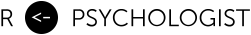# Understanding Maximum Likelihood

## An Interactive Visualization

Created by Kristoffer Magnusson

The maximum likelihood method is used to fit many models in statistics. In this post I will present some interactive visualizations to try to explain maximum likelihood estimation and some common hypotheses tests (the likelihood ratio test, Wald test, and Score test).

We will use a simple model with only two unknown parameters: the mean and variance. Our primary focus will be on the mean and we'll treat the variance as a nuisance parameter.

## Likelihood Calculation

Before we do any calculations, we need some data. So, here's 10 random observations from a normal distribution with unknown mean (μ) and variance (σ²).

Y = [1.0, 2.0]

We also need to assume a model, we're gonna go with the model that we know generated this data: $y \sim \mathcal N(\mu, \sigma^2)$. The challenge now is to find what combination of values for μ and σ² maximize the likelihood of observing this data (given our assumed model). Try moving the sliders around to see what happens.

Mean (μ)
Variance (σ²)

We can calculate the joint likelihood by multiplying the densities for all observations. However, often we calculate the log-likelihood instead, which is

$\ell(\mu, \sigma^2) = \sum_i^n \text{ln} \thinspace f_y(y_i)=$-34.4+-33.6=-68.1

The combination of parameter values that give the largest log-likelihood is the maximum likelihood estimates (MLEs).

## Finding the Maximum Likelihood Estimates

Since we use a very simple model, there's a couple of ways to find the MLEs. If we repeat the above calculation for a wide range of parameter values, we get the plots below. The joint MLEs can be found at the top of contour plot, which shows the likelihood function for a grid of parameter values. We can also find the MLEs analytically by using some calculus. We find the top of the hill by using the partial derivatives with regard to μ and σ² - which is generally called the score function (U). Solving the score equations mean that we find which combination of μ and σ² leads to both partial derivates being zero.

### Mean

Tip: You can move the values around my dragging them.

For more challenging models, we often need to use some optimization algorithm. Basically, we let the computer iteratively climb towards the top of the hill. You can use the controls below to see how a gradient ascent or Newton-Raphson algorithm finds it's way to the maximum likelihood estimate.

Iterations: 0

## Inference

After we've found the MLEs we usually want to make some inferences, so let's focus on three common hypothesis tests. Use the sliders below to change the null hypothesis and the sample size.

## Illustration

Sample Size (n)
Null (μ0)

The score function evaluated at the null is,

$U(\mu_0, \hat\sigma_0^2) = \frac{\partial}{\partial \mu_0}\ell(\mu_0, \hat\sigma_0^2) = -Infinity$

The observed Fisher information is the negative of the second derivative. This is related to the curvature of the likelihood function -- try increasing the sample size and note that the peak gets narrower around the MLE and that the information increases. The inverse of I is also the variance of the MLE.

$I(\mu_0, \hat\sigma_0^2) = \frac{\partial^2}{\partial \mu_0^2}\ell(\mu_0, \hat\sigma_0^2) = Infinity$

## Hypothesis Tests

We have the following null and alternative hypothesis,

$H_0: \mu = 80 \quad \text{versus} \quad H_1: \mu \ne 80$

The likelihood ratio test compares the likelihood ratios of two models. In this example it's the likelihood evaluated at the MLE and at the null. This is illustrated in the plot by the vertical distance between the two horizontal lines. If we multiply the difference in log-likelihood by -2 we get the statistic,

\begin{aligned} \text{LR} &= -2[\ell(\mu_{0}, \hat\sigma^2_{0}) - [\ell(\hat\mu, \hat\sigma^2)]\\ &= NaN \end{aligned}

Asymptotically LR follow a$\chi^2$ distribution with 1 degrees of freedom, which gives p = NaN.

Note: The figure is simplified and do not account for the fact that each likelihood is based on different variance estimates.

## FAQ

Cite this page according to your favorite style guide. The page is created by Kristoffer Magnusson, and you can find the current version number and the date of the last update in the footer.